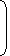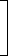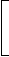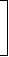# Problem solving tricks on sales and discountIMPORTANT CONCEPTS

Suppose a man has to pay Rs. 156 after 4 years and the rate of interest is 14% per annum. Clearly, Rs. 100 at 14% will amount to R. 156 in 4 years. So, the payment of Rs. now will clear off the debt of Rs. 156 due 4 years hence. We say that:

Sum due = Rs. 156 due 4 years hence;

Present Worth (P.W.) = Rs. 100;

True Discount (T.D.) = Rs. (156 – 100) = Rs. 56 = (Sum due) – (P.W.)

We define: T.D. = Interest on P.W.;     Amount = (P.W.) + (T.D.)

Interest is reckoned on P.W. and true discount is reckoned on the amount.

IMPORTANT FORMULAE

Let rate = R% per annum and Time = T years. Then,

 1.   P.W. = 100 x Amount = 100 x T.D. 100 + (R x T) R x T
 2.   T.D. = (P.W.) x R x T = Amount x R x T 100 100 + (R x T)
 3.   Sum = (S.I.) x (T.D.) (S.I.) – (T.D.)

4.   (S.I.) – (T.D.) = S.I. on T.D.

5.   When the sum is put at compound interest, then P.W. = Amount1 + RT 100
A man purchased a cow for Rs. 3000 and sold it the same day for Rs. 3600, allowing the buyer a credit of 2 years. If the rate of interest be 10% per annum, then the man has a gain of:
 A. 0% B. 5% C. 7.5% D. 10%

Answer: Option A

Explanation:

C.P. = Rs. 3000.

 S.P. = Rs.3600 x 100= Rs. 3000. 100 + (10 x 2)

Gain = 0%.

A trader owes a merchant Rs. 10,028 due 1 year hence. The trader wants to settle the account after 3 months. If the rate of interest 12% per annum, how much cash should he pay?
 A. Rs. 9025.20 B. Rs. 9200 C. Rs. 9600 D. Rs. 9560

Answer: Option B

Explanation:

Required money = P.W. of Rs. 10028 due 9 months hence
= Rs.10028 x 100100 +12 x 912
= Rs. 9200.

Correct! Wrong!

Correct! Wrong!

Correct! Wrong!

Correct! Wrong!

#### The present worth of Rs. 1404 due in two equal half-yearly installments at 8% per annum simple interest is:

Correct! Wrong!

QUIZ ON TRUE DISCOUNT
CONGO!!!

Share your Results:

Don't miss out!
Subscribe To Our Newsletter

Learn new things. Get an article everyday.

Invalid email address
Give it a try. You can unsubscribe at any time.